## GeomArcbar

`geom_arcbar` plots arc bar diagrams that span 180 degrees. They also take an optional spacing argument `sep` that is entered as a total proportion of pi. This is best demonstrated with an example:

``````bt <- data.frame(
parties = factor(c("CDU", "CSU", "AfD", "FDP", "SPD",
levels = c("CDU", "CSU", "AfD", "FDP", "SPD",
seats   = c(200, 46, 92, 80, 153, 69, 67, 2),
colors  = c("black", "blue", "lightblue", "yellow", "red",
"purple", "green", "grey"),
stringsAsFactors = FALSE)

ggplot(bt) +
geom_arcbar(aes(shares = seats, r0 = 5, r1 = 10, fill = parties),
sep = 0.1) +
scale_fill_manual(values = bt\$colors) +
coord_fixed() +
theme_void()``````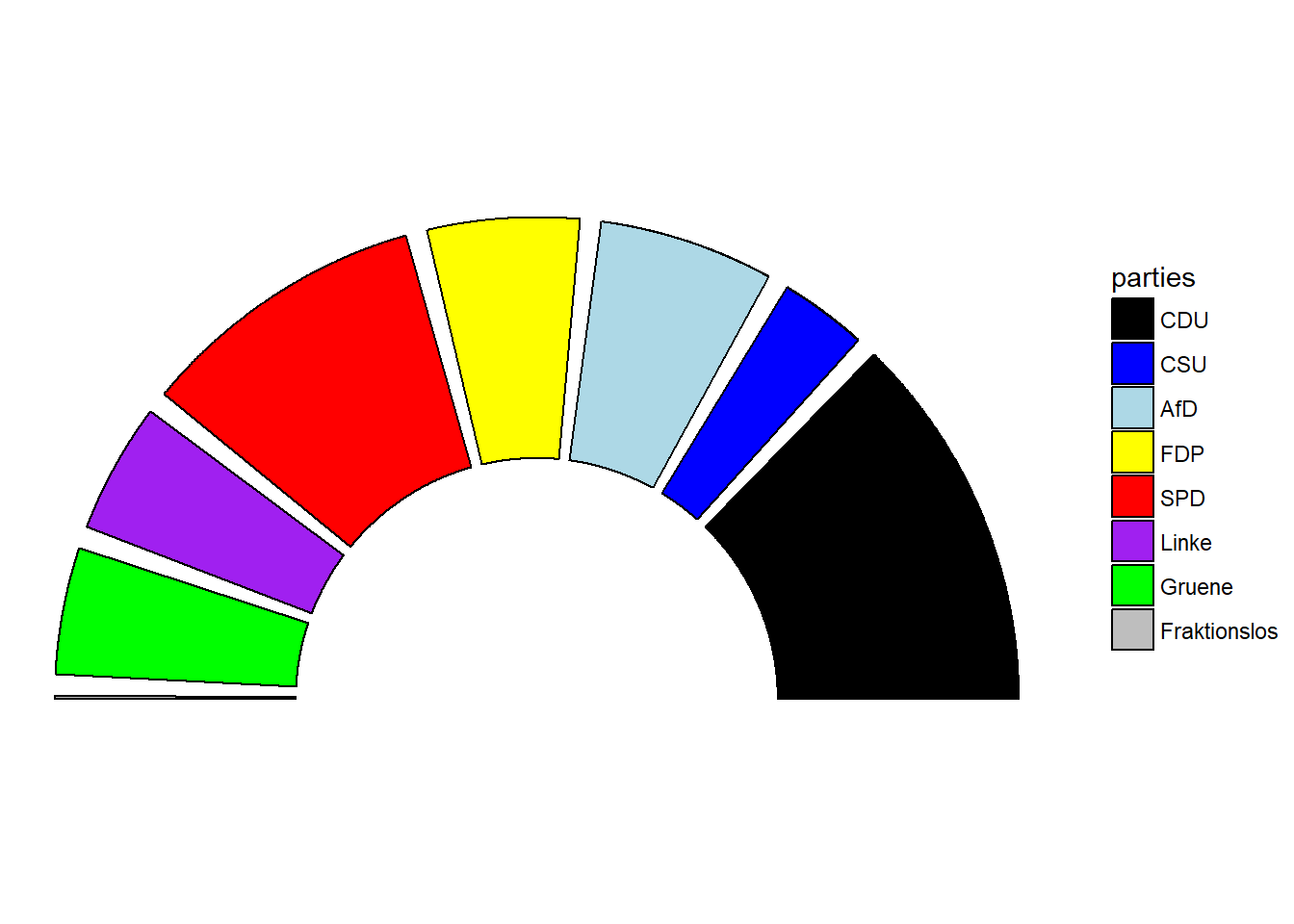## GeomBartext

`geom_bartext` is a Stackoverflow-inspired, experimental feature (and currently only available via the developer version) for unstacking overlapping text-labels in barcharts. If overlapping, `geom_bartext` will repel all groups of overlapping labels vertically or horizontally to make for a readable graph.

Consider e.g. this:

``````df <- data.frame(L = rep(LETTERS[1:2], each = 4),
l = rep(letters[1:4], 2),
val = c(96.5, 1, 2, 0.5, 48, 0.7, 0.3, 51))

gridExtra::grid.arrange(
ggplot(df, aes(x = L, y = val, fill = l)) +
geom_bar(stat = "identity") +
geom_text(aes(label = scales::percent(val / 100)), position = position_stack(vjust = 0.5)) +
ggtitle("GeomText"),
ggplot(df, aes(x = L, y = val, fill = l)) +
geom_bar(stat = "identity") +
geom_bartext(aes(label = scales::percent(val / 100)), position = position_stack(vjust = 0.5)) +
ggtitle("GeomBartext"),
ncol = 2
)``````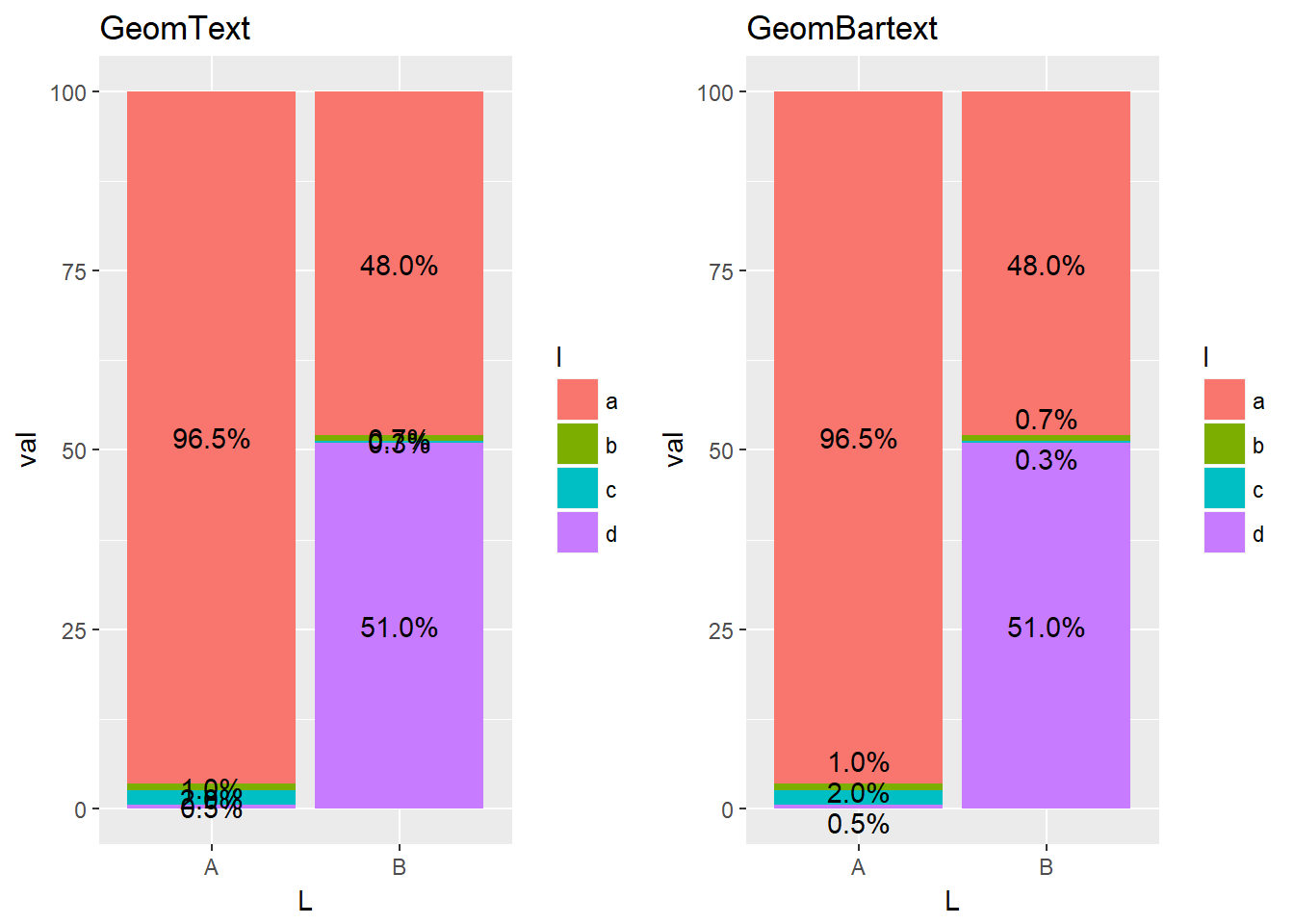`geom_bartext` takes all the arguments you would usually pass to `geom_text`, plus the `dir` argument which can be set to either `v` (vertical, default) or `h` (horizontal) repel.

Sometimes you might want additional spacing between the labels. In that case you can specify the `spacing` argument, which is set to `0.003` default (`npc` units).

## GeomParliament

`geom_parliament` plots parliament diagrams, i.e. circles on an arc, where each circle represents a single Member of Parliament. If `fill` or `color` is specified, the points will be clustered by party (or rather, the `seats` argument).

``````bt <- data.frame(
parties = factor(c("CDU", "CSU", "AfD", "FDP", "SPD",
levels = c("CDU", "CSU", "AfD", "FDP", "SPD",
seats   = c(200, 46, 92, 80, 153, 69, 67, 2),
colors  = c("black", "blue", "lightblue", "yellow",
"red","purple", "green", "grey"),
stringsAsFactors = FALSE)

ggplot(bt) +
geom_parliament(aes(seats = seats, fill = parties), color = "black") +
scale_fill_manual(values = bt\$colors, labels = bt\$parties) +
coord_fixed() +
theme_void()``````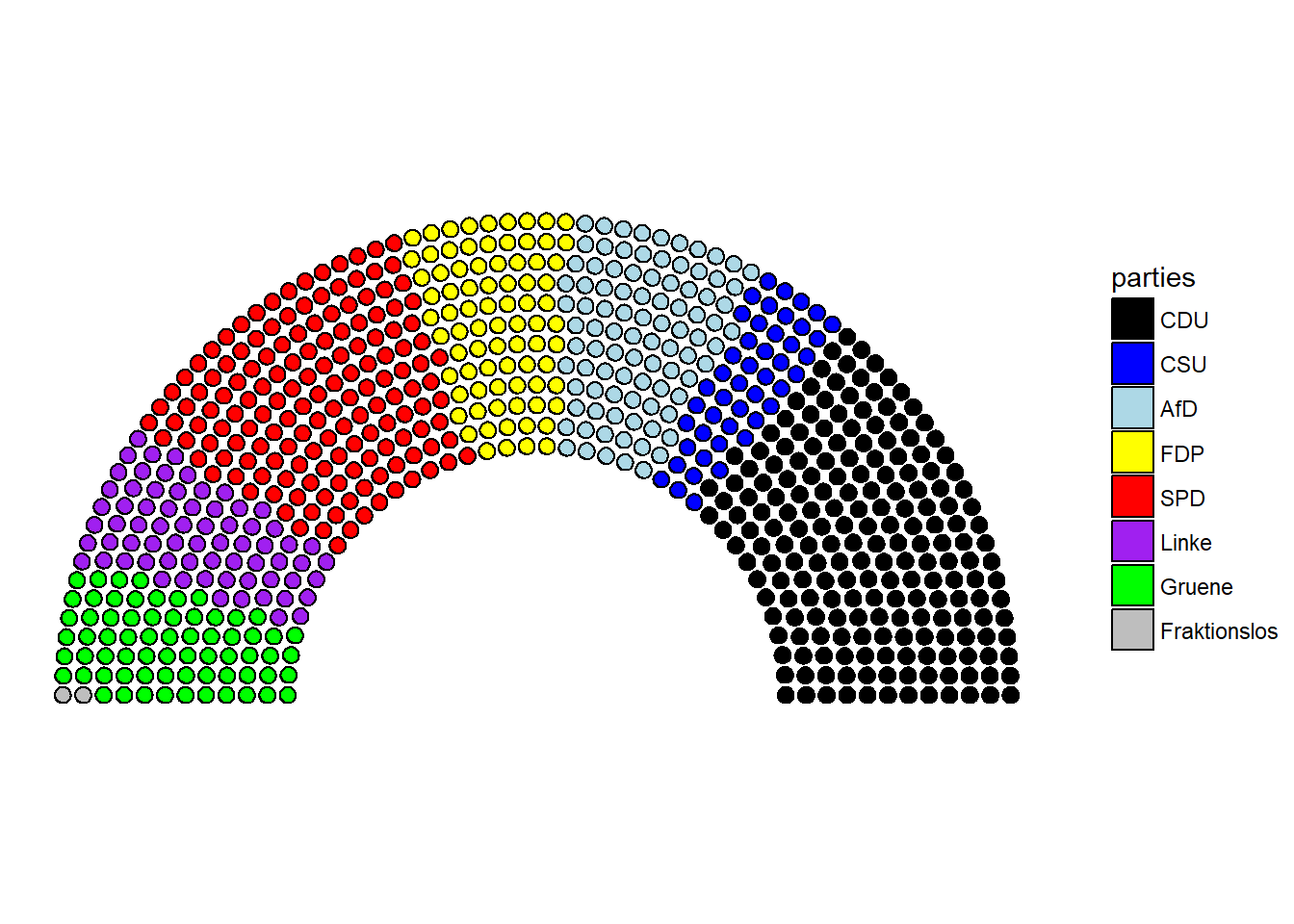Currently, the grouping is always calculated using the `seats` argument.

## GeomCircle

`geom_circle` plots circle-polygons with a specified radius, rather than `size` in `geom_point`. As such, this is also by default used by `geom_parliament`.

``````df <- data.frame(x = sample(1:10, 3), y = sample(1:10, 3),
r = sample(3:4, 3, replace = TRUE))

ggplot(df) + geom_circle(aes(x = x, y = y, r = r, fill = gl(3, 1))) +
coord_fixed()``````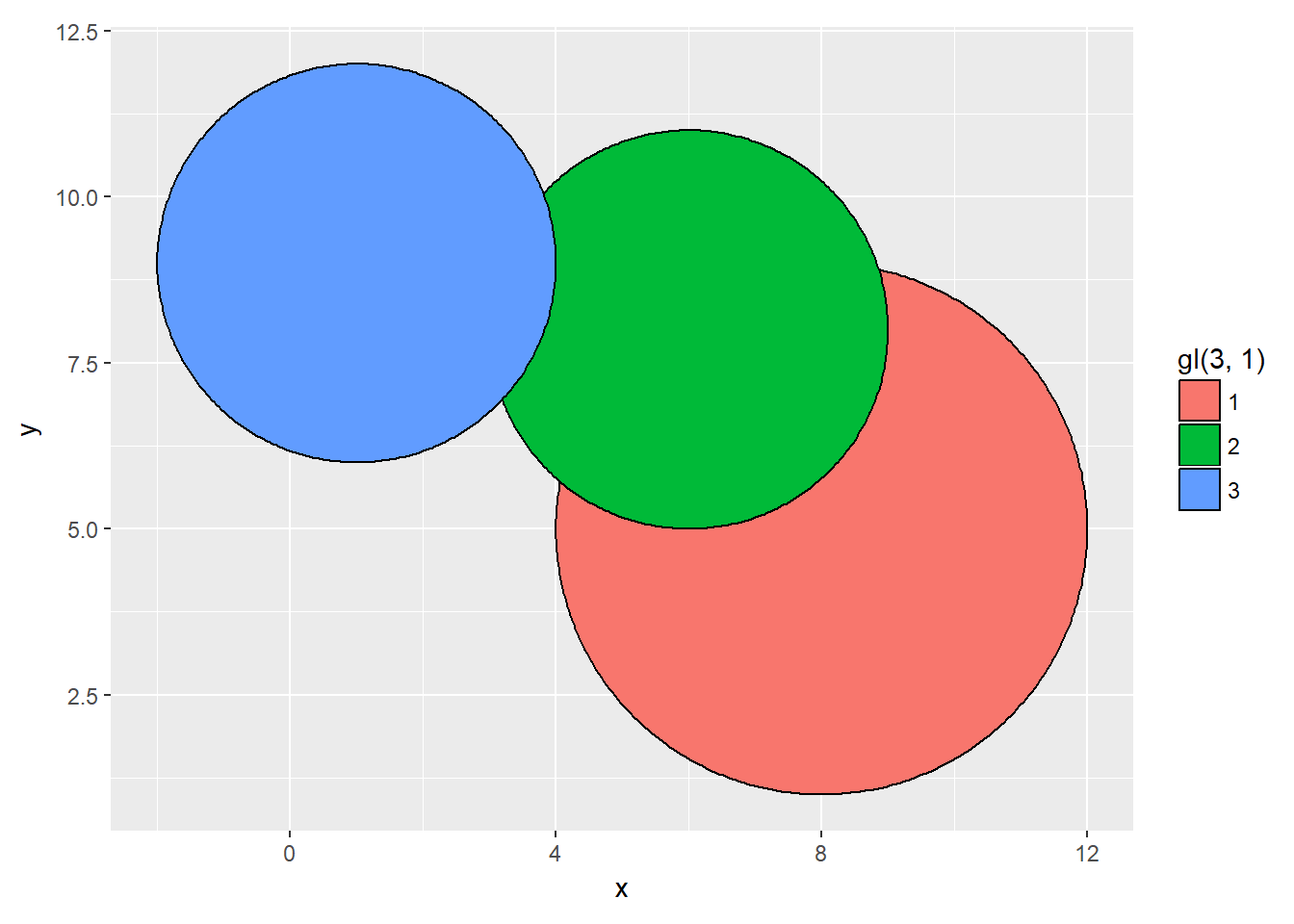## GeomTshighlight

`geom_tshighlight` (for timeseries-highlight) is a wrapper around `geom_rect` that uses default values for `ymin` and `ymax` so that it spans the entire y-axis.

``````ggplot(economics, aes(x = date, y = unemploy)) +
geom_line() +
geom_tshighlight(aes(xmin = as.Date("01/01/1990", format = "%d/%m/%Y"),
xmax = as.Date("01/01/2000", format = "%d/%m/%Y")),
alpha = 0.01)``````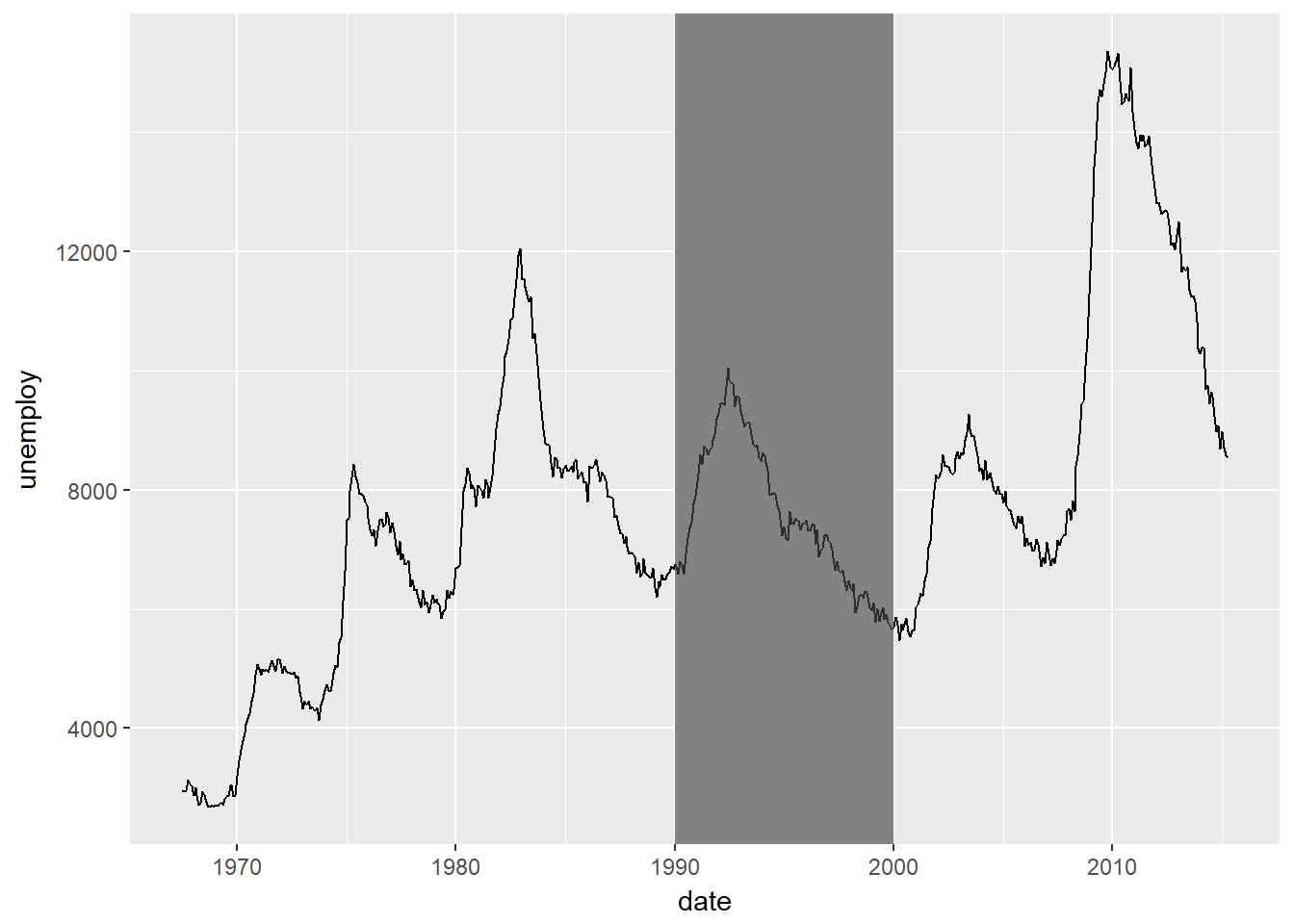## GeomBoxjitter

`geom_boxjitter` plots a hybrid boxplot - half boxplot, half scatterplot - with optional errorbars. The jitter is customizable vertically and horizontally and also supports a `seed` argument. Note that, if you specify e.g. `fill` for the boxplot, you need to select a `jitter.shape` that actually supports this. Shapes 21-25 support fill, and 21 is a circle.

``````df <- data.frame(score = rgamma(150, 4, 1),
gender = sample(c("M", "F"), 150, replace = TRUE),
genotype = factor(sample(1:3, 150, replace = TRUE)))

ggplot(df) +
geom_boxjitter(aes(x = genotype, y = score, fill = gender),
jitter.shape = 21, jitter.color = NA,
jitter.height = 0, jitter.width = 0.04,
outlier.color = NA, errorbar.draw = TRUE) +
scale_fill_manual(values = c("#ecb21e", "#812e91")) +
theme_minimal()``````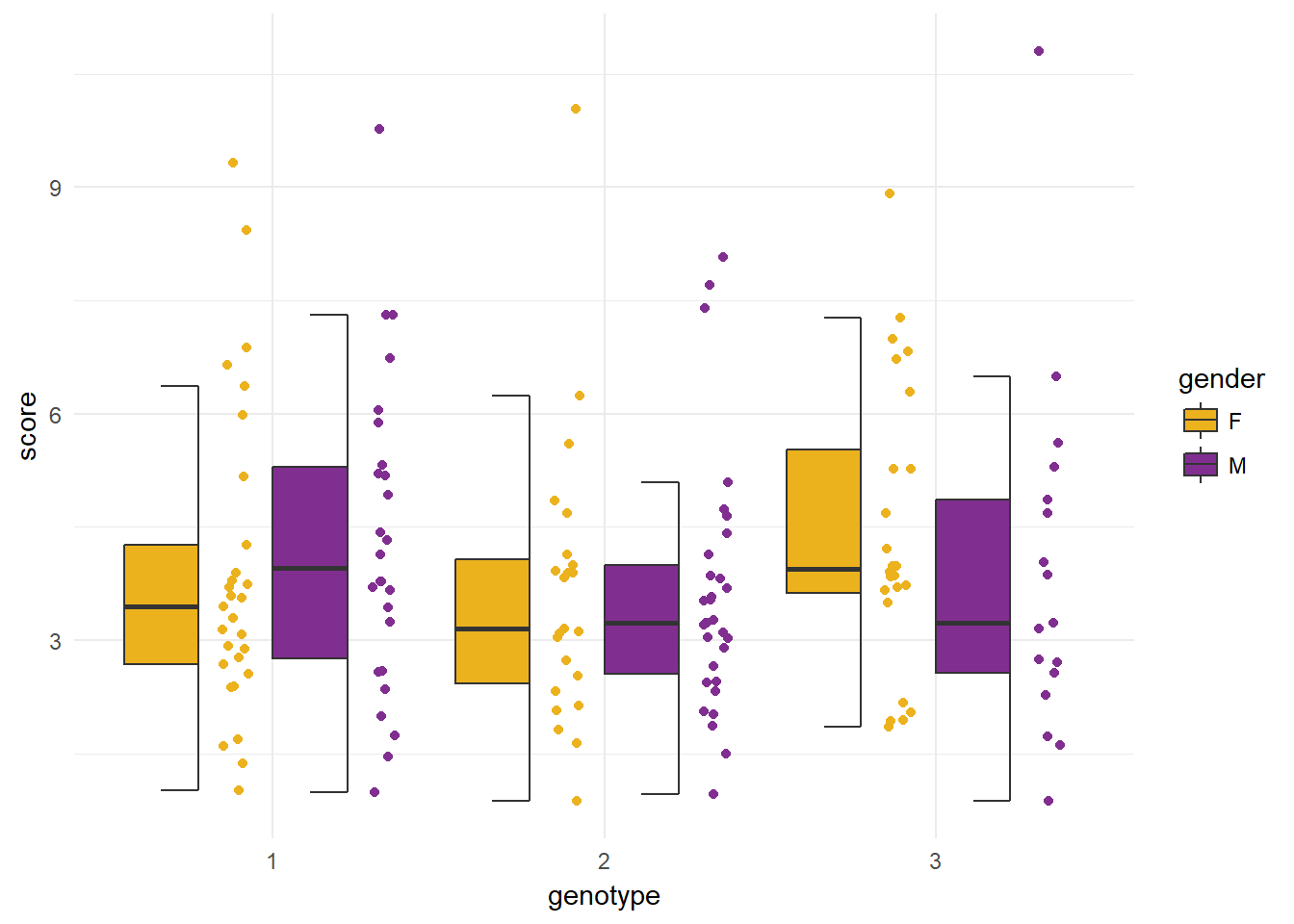By default, outliers are a normal part of the jitterplot. We might want to highlight them, which can be done by setting `outlier.intersect` to `TRUE`. We also select a different outlier shape to clearly differentiate them from the scatter points.

``````ggplot(df) +
geom_boxjitter(aes(x = genotype, y = score, fill = gender),
jitter.shape = 21, jitter.color = NA,
jitter.height = 0, jitter.width = 0.04,
outlier.color = "black", errorbar.draw = TRUE,
outlier.intersect = TRUE, outlier.shape = 24,
outlier.size = 1.5) +
scale_fill_manual(values = c("#ecb21e", "#812e91")) +
theme_minimal()``````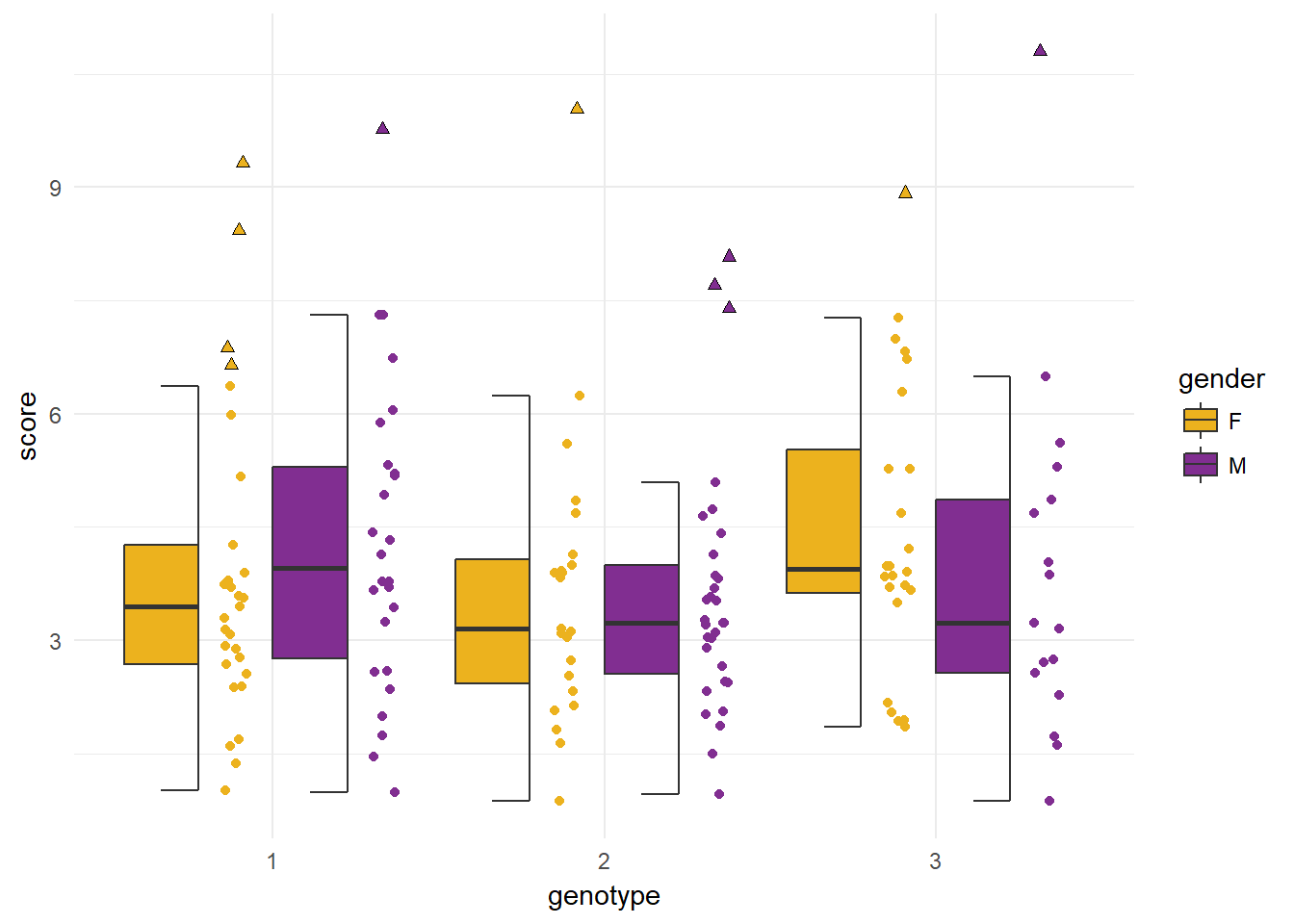It is also possible to hide the jitter altogether by setting `boxplot.expand` to `TRUE`.. In that case, `geom_boxjitter` is equal to `geom_boxplot`, but has additional, configurable errorbars.

``````ggplot(df) +
geom_boxjitter(aes(x = genotype, y = score, fill = gender),
errorbar.draw = TRUE, boxplot.expand = TRUE,
errorbar.length = 0.4) +
scale_fill_manual(values = c("#ecb21e", "#812e91")) +
theme_minimal()``````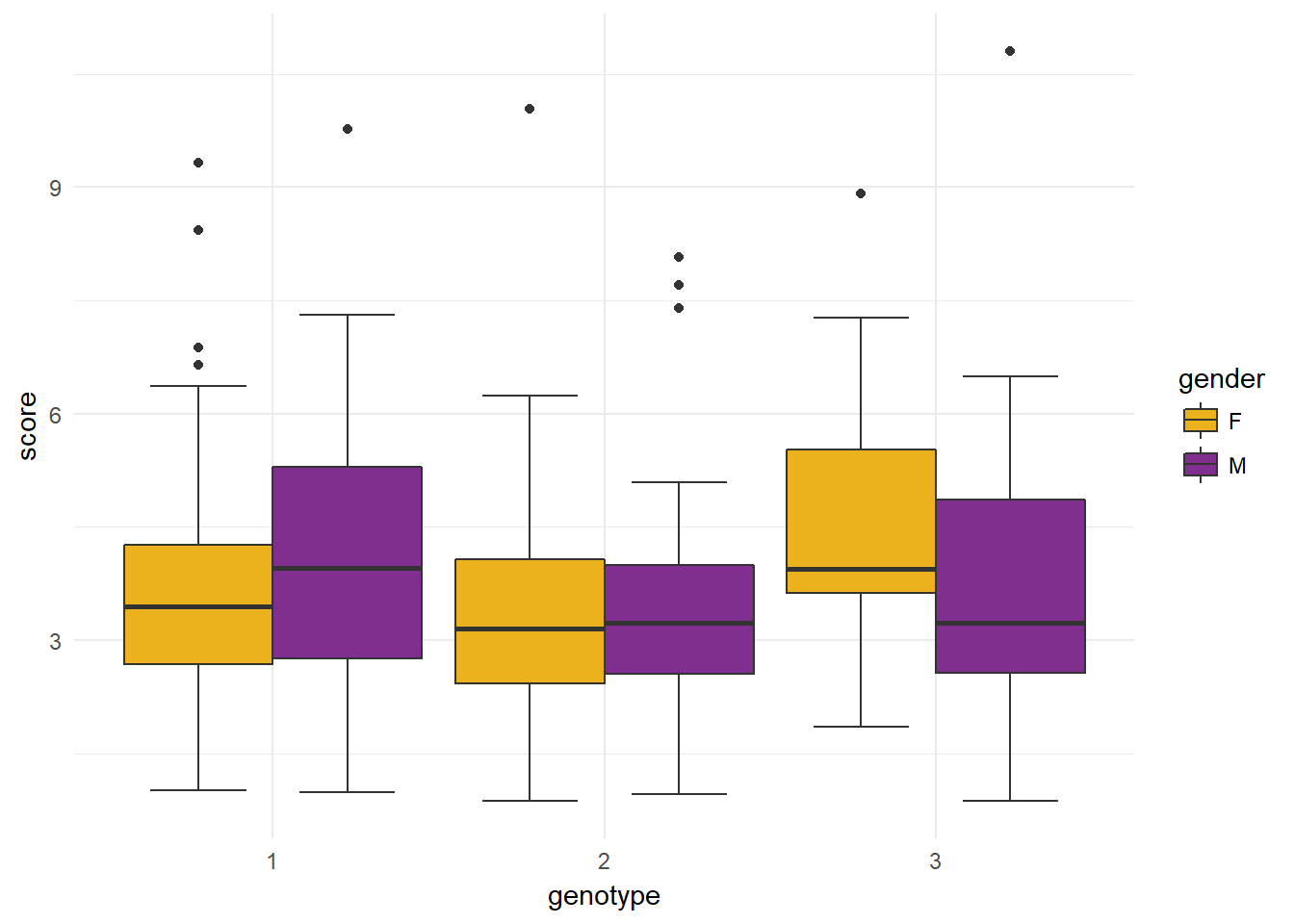## FacetShare

`facet_share` is an experimental feature that implements basic shared axes for `FacetWrap` with only two panels (i.e., this will not work if you stratify by a variable with more than 2 levels). If we want to mirror our axis, we will have to multiply one panel by -1 (the choice of which panel to multiply by -1 is natural, i.e. the left one for horizontal, and the bottom one for vertical directions). Since this will also change the axis labels for that panel, setting `reverse_num` to `TRUE` will reverse this change.

The horizontal mirroring also requires using `coord_flip()`.

Naturally, the shared axis is well suited for population pyramids.

``````df <- data.frame(sex = sample(c("M", "F"), 1000, replace = TRUE),
age = rnorm(1000, 45, 12))

df\$age_bins <- cut(df\$age, 15)
df\$count <- 1
df <- aggregate(count ~ sex + age_bins, data = df, length)

df_h <- df
df_h\$count <- ifelse(df_h\$sex == "F", df_h\$count * -1, df_h\$count)

ggplot(df_h, aes(x = age_bins, y = count, fill = sex)) +
geom_bar(stat = "identity") +
facet_share(~sex, dir = "h", scales = "free", reverse_num = TRUE) +
coord_flip()``````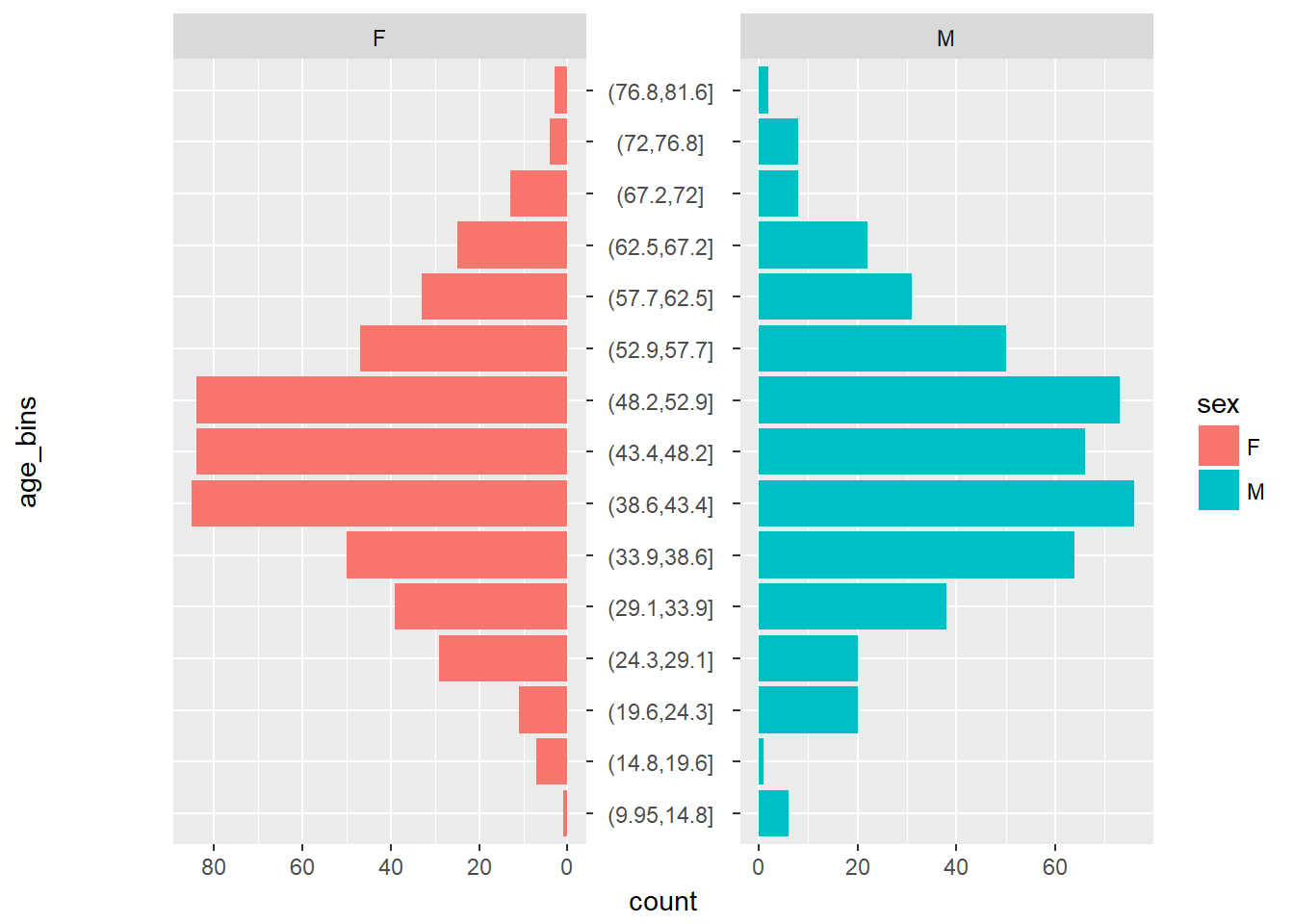And vertically:

``````# When setting direction to vertical, and if we want to mirror the second panel,
# we must multiply the second factor by -1.
# And levels(factor(gender)) is M.
df_v <- df
df_v\$count <- ifelse(df_v\$sex == "M", df_v\$count * -1, df_v\$count)
ggplot(df_v, aes(x = age_bins, y = count, fill = sex)) +
geom_bar(stat = "identity") +
facet_share(~sex, dir = "v", scales = "free", reverse_num = TRUE)``````